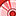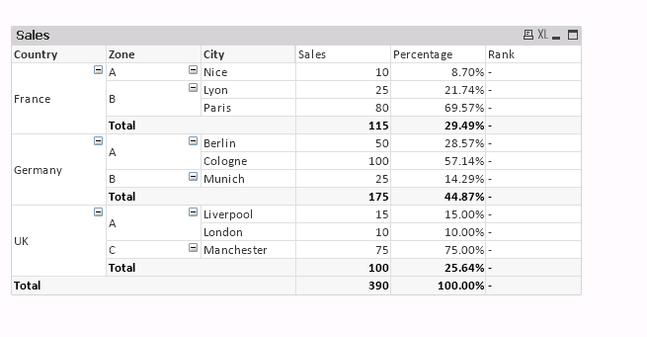Announcements
You can succeed best and quickest by helping others to succeed. Join the conversation.
cancel
Showing results for
Did you mean:Contributor II

## Rank on SubTotal with respect to Grand total

Hello ,

Country -France , Rank of City would be Paris = 1,Lyon=2, Nice=3

but SubTotal rank would be 2 as it is 29.49% according to Grand total.

I have attached the documentLabels (1)
• ### Rank on SubTotal with respect to Grand total

1 Solution

Accepted SolutionsMVP

Try like below

If(RowNo()=0, Aggr(Rank(Sum(TOTAL<Country> [Count of Sales])), Country), Aggr(Rank(Sum( [Count of Sales]),4,1),Country, City))

output:Thanks & Regards, Mayil Vahanan RMVP

Try like below

If(RowNo()=0, Aggr(Rank(Sum(TOTAL<Country> [Count of Sales])), Country), Aggr(Rank(Sum( [Count of Sales]),4,1),Country, City))

output:Thanks & Regards, Mayil Vahanan R